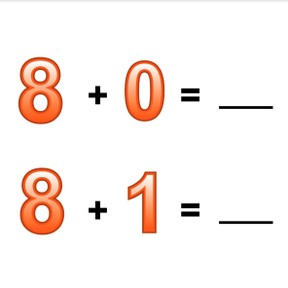Addition to 10 with one more, one less

# Addition to 10 with one more, one less

I can add up to 10 with one more or one less.8,000 schools use Gynzy92,000 teachers use Gynzy1,600,000 students use Gynzy

## General

Students learn to add to 10 in which they add one more, or one less.

K.OA.A.2

## Introduction

Hand out number cards from 1 to 10 to the students. Every student has a number card. As a class, count from 1 to 10. When the students hear the number that is on their number card, they must stand up. Next start counting from the number 4. Have the students exchange number cards so they have a new number card. Write a number on the interactive whiteboard. If a student's number card is one before or one after the number you wrote down. Repeat this with all the numbers.

## Instruction

To check that students understand adding to 10 with one more and one less you can ask them the following questions/give the following exercises:
- What do you look at to know if you need to count one more or one less?
- Calculate: 5+3= and 5+4
- Calculate: 7+2= and 6+2

## Quiz

Students first practice adding one more or one less with a set of two problems. They then practice with a set of 3 problems.

## Closing

Discuss with students that it is important to be able to add to 10 with one more and one less, so you can quickly solve sets of problems without needing to calculate each one separately. Show some problems on the interactive whiteboard and ask students if they can calculate them without doing each problem separately. Ask students how they got their answers and if there are any ways to do the problems in an easier or faster way, or if they solved each problem separately. Ask students to work in pairs. One student writes down a math problem. The second student writes a similar problem, with one more or one less. They solve the problems together.

## Teaching tips

Students who have difficulty adding with one more and one less can make use of manipulatives like MAB blocks. Have them set out blocks or manipulatives to represent the math problems and then add one block or take one away.

## Instruction materials

Number cards to 10, MAB blocks or other manipulatives

### The online teaching platform for interactive whiteboards and displays in schools

• Save time building lessons

• Manage the classroom more efficiently

• Increase student engagement